# Addition And Subtraction Of Complex Numbers

Go back to  'Complex Numbers'

Consider two numbers $${z_1} = {x_1} + i\,{y_1}\,\,\,{\rm{and}}\,\,{z_2} = \,\,{x_2}\, + \,\,i\,{y_2}$$ which we have to add/subtract.

For any complex number, since the real and imaginary components are along mutually perpendicular directions, this suggests that to add/subtract two complex numbers, we add/subtract their real and imaginary components separately.

That is,

${z_1} \pm {z_2} = \left( {{x_1} + {x_2}} \right) \pm i\left( {{y_1} + {y_2}} \right)\;\;\;\;\;\;\;\;...(1)$

This definition of addition/subtraction is intuitively appealing.

How is this definition consistent with the way we understand complex numbers graphically?

Notice that (1) simply suggests that complex numbers add/subtract like vectors. The two mutually perpendicular components add/subtract separately. Therefore, our graphical interpretation of complex numbers is further validated by this approach (vector approach) to addition / subtraction.

To actually carry out addition / subtraction, we simply use the parallelogram law.

$\fbox{$${{\rm{Addition}}\,\,\,\left( {{z_1} + {z_2}} \right)}$$}$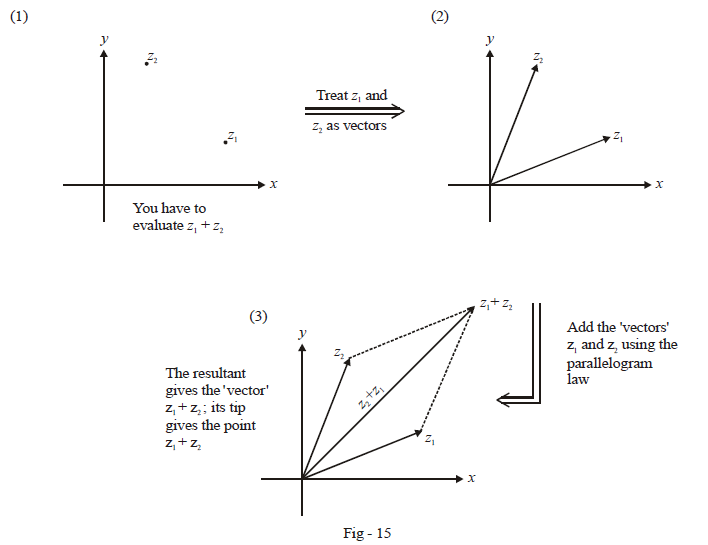$\fbox{$${{\text{Subtraction}}\,\,\,\left( {{z_1} - {z_2}} \right)}$$}$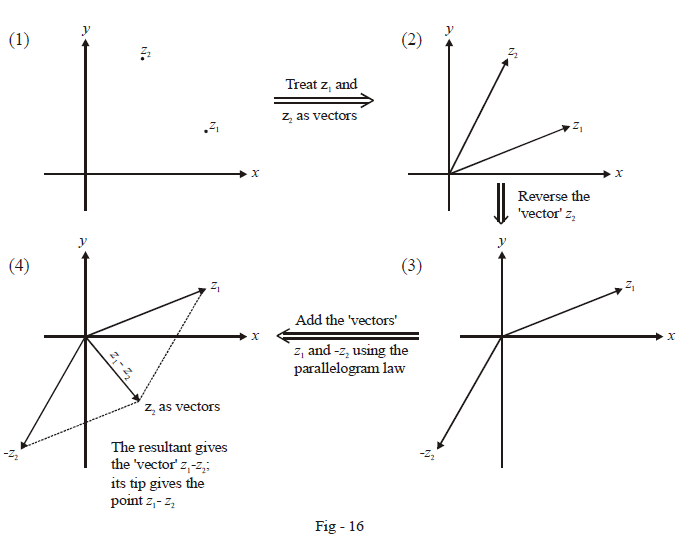Notice that we have made a distinction between, for example, the vector $${z_1} + {z_2}$$  and the point $${z_1} + {z_2}$$; similarly, a distinction has been made between the vector $${z_1} - {z_2}$$  and the point $${z_1} - {z_2}$$  . You must understand this distinction carefully.

A vector is a free entity. It will remain the same as long as it is translated without rotation anywhere in the plane. So, for example, the vector$${z_1} + {z_2}$$, as long as it maintains its magnitude and direction, can lie anywhere in the plane; it will still remain the vector $${z_1} + {z_2}$$ :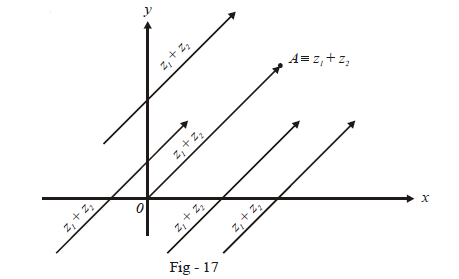However, to determine the point $${z_1} + {z_2}$$  in the plane, we must make the tail end-point of the vector  $${z_1} + {z_2}$$ co-incide with the origin; the tip of this vector then gives the point $${z_1} + {z_2}$$ . For example, the tip $$A$$ of the vector $$\overrightarrow {OA}$$ in the figure above gives the point  $${z_1} + \,\,{z_2}$$ .

This distinction will mean that the triangle law when applied to the subtraction problem will give us the vector $${z_1} - \,\,{z_2}$$  and not the point $${z_1} - \,\,{z_2}$$ .  To determine the point $${z_1} - \,\,{z_2}$$, we make the tail end point of the vector $${z_1} - \,\,{z_2}$$ co-incide with the origin.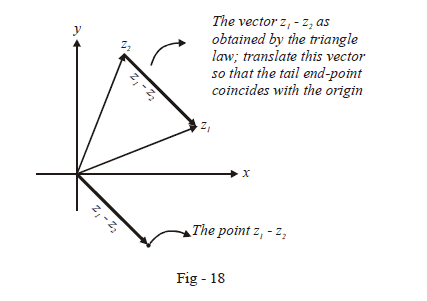Before concluding, observe that $$\left| {{z_1} - \,\,{z_2}} \right|$$ will represent the length of the vector $${z_1} - \,\,{z_2}$$  or the distance between the points $$z_1$$ and $$z_2.$$ This is extremely important: the expression $$| a – b |$$should always be interpreted as the distance between the points $$a$$ and $$b.$$

Example- 2

Evaluate the expression for  $${\left| {{z_1} \pm \,\,{z_2}} \right|^2}$$ using the polar forms for $$z_1$$ and $$z_1.$$

Solution: Let $${z_1} = {r_1}\cos {\theta _1} + i\,{r_1}\sin {\theta _1}$$  and   $${z_2} = {r_2}\cos {\theta _2} + i\,{r_2}\sin {\theta _2}$$

\begin{align}\Rightarrow \qquad {z_1}\,\, \pm \,\,{z_2} &= \left( {{r_1}\cos {\theta _1}\,\, \pm \,\,{r_2}\cos {\theta _2}} \right) + i\left( {{r_1}\sin {\theta _1}\,\, \pm \,\,{r_2}\sin {\theta _2}} \right)\\\\\Rightarrow \quad {\left| {{z_1}\,\, \pm \,\,{z_2}} \right|^2} &= {\left( {{r_1}\cos {\theta _1}\,\, \pm \,\,{r_2}\cos {\theta _2}} \right)^2} + {\left( {{r_1}\sin {\theta _1}\,\, \pm \,\,{r_2}\sin {\theta _2}} \right)^2}\\\\&= r_1^2 + r_2^2\,\, \pm \,\,2{r_1}\,{r_2}\cos \left( {{\theta _1} - {\theta _2}} \right)\end{align}

Example- 3

Carry out the following operations graphically:

(a) $$\left( {2 + i} \right) + \left( {3 - i} \right)$$                   (b) $$\left( {2 - 2i} \right) + \left( { - 2 + 3i} \right)$$

(c) $$\left( {3 - i} \right) - \left( {2 - i} \right)$$                    (d) $$\left( 3 \right) - \left( {5 + 2i} \right)$$

Solution: Observe carefully how the parallelogram law is applied to each of the four parts: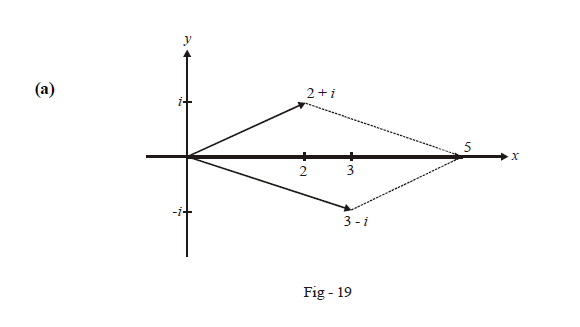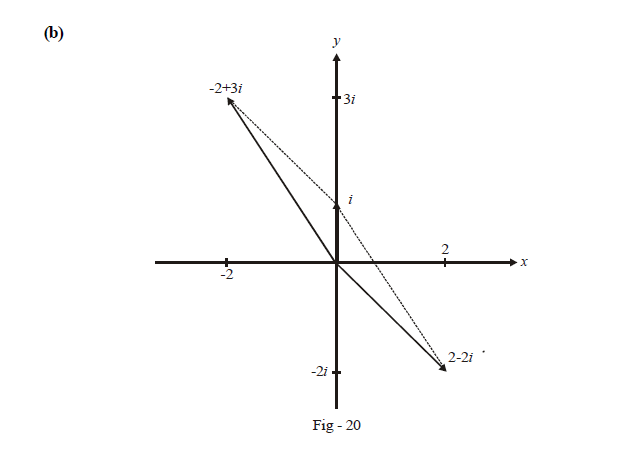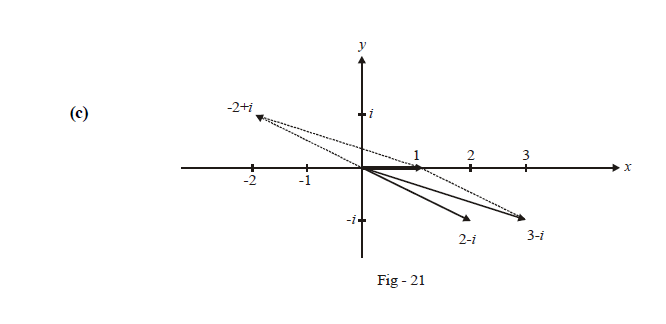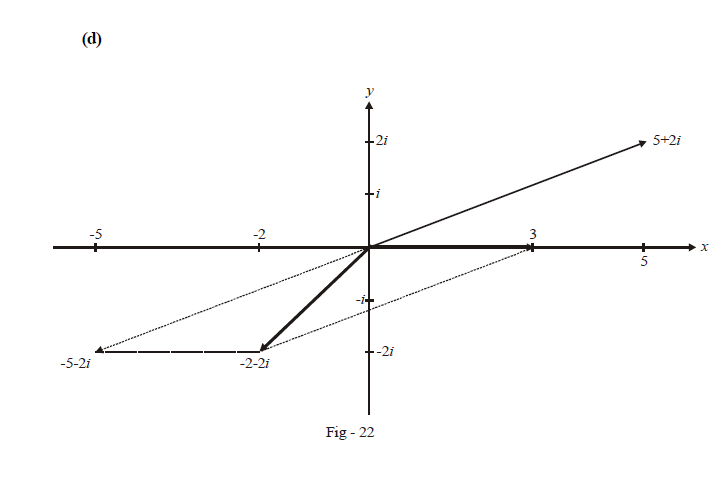Example- 4

Prove the Triangle Inequality:

For any two arbitrary complex numbers $$z_1$$ and $$z_2,$$

$\left| {\left| {{z_1}} \right| - \left| {{z_2}} \right|} \right|\,\, \le \,\,\left| {{z_1} + {z_2}} \right|\,\, \le \,\,\left| {{z_1}} \right| + \left| {{z_2}} \right|$

Solution: From example-2, observe that

${\left| {{z_1} + {z_2}} \right|^2} = r_1^2 + r_2^2 + 2{r_1}\,{r_2}\,\,\cos \left( {{\theta _1} - {\theta _2}} \right)$

Now, $$- 1\,\, \le \,\,\cos \left( {{\theta _1} - {\theta _2}} \right)\,\, \le \,\,1$$

\begin{align}&\Rightarrow r_1^2 + r_2^2 - 2{r_1}\,{r_2}\,\, \le \,\,{\left| {{z_1} + {z_2}} \right|^2}\,\, \le \,\,r_1^2 + r_2^2 + 2{r_1}\,{r_2}\\&\Rightarrow {\left( {{r_1} - {r_2}} \right)^2} \le {\left| {{z_1} + {z_2}} \right|^2} \le {\left( {{r_1} + {r_2}} \right)^2}\\ &\text{or}\; {\left( {\left| {{z_1}} \right| - \left| {{z_2}} \right|} \right)^2}\,\, \le \,\,{\left| {{z_1} + {z_2}} \right|^2}\,\, \le \,\,{\left( {\left| {{z_1}} \right| + \left| {{z_2}} \right|} \right)^2}\end{align}

Taking the square root for all the three terms, we get:

$\left| {\left| {{z_1}} \right| - \left| {{z_2}} \right|} \right|\,\, \le \,\,\left| {{z_1} + {z_2}} \right|\,\, \le \,\,\left| {{z_1}} \right| + \left| {{z_2}} \right|$

Let us understand this inequality geometrically, which will be much more illuminating . Consider a triangle with sides represented by (vectors) $${z_1},\;{z_2}$$ and $${z_1} + {z_2}$$ :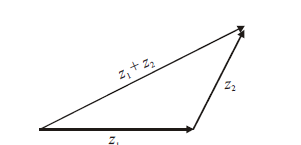We use the geometrical fact that in a triangle, the sum of two sides is greater than the third side:

$|{z_1}| + |{z_2}|\; > \;|{z_1} + {z_2}|$

On the other hands, the difference of two sides is smaller than the third side:

$\left| {|{z_1}| - |{z_2}|} \right|\; < \;|{z_1} + {z_2}|$

However, in both the above cases, equality may hold, in the cases that $${z_1}\;{\rm{and}}\;{z_2}$$  are parallel or anti - parallel respectively: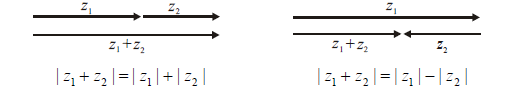Combining all these facts, we obtain the complete triangle inequality.

TRY YOURSELF - II

Q. 1  This question is for you to practice addition and subtraction  of complex numbers graphically. In each case, you are expected to perform the indicated operations graphically on the Argand plane.

 (a)  $$\left( {2 + i} \right) + \left( {3 + 2i} \right)$$ (b)  $$\left( { - 1 - i} \right) + \left( {6 + 2i} \right)$$ (c)  $$\left( {7i - 2} \right) - \left( {3i + 4} \right)$$ (d)  $$\left( { - 6} \right) - \left( {3 - i} \right)$$ (e)  $$\left( {1 + i} \right) + \left( {2 + i} \right) + \left( {3 + i} \right)$$ (f)   \begin{align}(1) + \left( { - \frac{1}{2} + \frac{{i\sqrt 3 }}{2}} \right) + \left( { - \frac{1}{2} - \frac{{i\sqrt 3 }}{2}} \right)\end{align}

Q. 2  Evaluate $$\arg ({z_1}) - \arg ({z_2})$$ if

(a)  $$|{z_1} + {z_2}|\; = \;|{z_1}| + |{z_2}|$$ .

(b)  $$|{z_1} + {z_2}|\; = \;|{z_1}| - |{z_2}|$$

(c)  $$|{z_1} + {z_2}{|^2} = \;|{z_1}{|^2} + |{z_2}{|^2}$$

Q. 3  If  $$|z - 2i|\; < \;\sqrt 2$$  ,  show that $$|i(z - 1) + 3|\; < \;2\sqrt 2$$

Learn from the best math teachers and top your exams

• Live one on one classroom and doubt clearing
• Practice worksheets in and after class for conceptual clarity
• Personalized curriculum to keep up with school